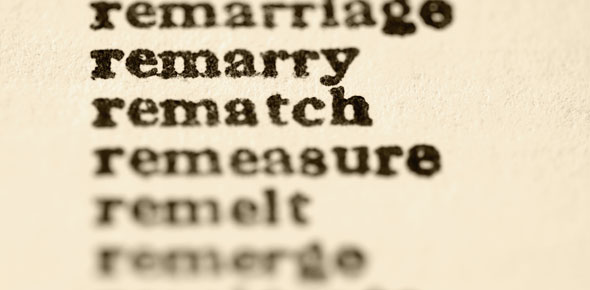# Vocabulary Quiz - Variables And Patterns

12 Questions | Total Attempts: 94SettingsTo all the current English students out there who are almost doing their exams, practice of the language is what makes all the difference. Take this quiz and gauge your proficiency.

• 1.
To become different
• A.

Variable

• B.

Rule

• C.

Change

• D.

Dependent variable

• 2.
A representation of data
• A.

Table

• B.

Pattern

• C.

Axis

• D.

Vertical

• 3.
A summary of predictable data that tells how to find the next value
• A.

X - axis

• B.

Y - axis

• C.

Change

• D.

Rule

• 4.
The horizontal line on a coordinate graph
• A.

X - axis

• B.

Y - axis

• C.

• D.

Diagonal

• 5.
A quantity that changes or varies
• A.

Axis

• B.

• C.

Variable

• D.

Table

• 6.
The vertical axis on a coordinate graph
• A.

X - axis

• B.

Y - axis

• C.

• D.

Variable

• 7.
A graph that shows the relationship between two variables
• A.

Coordinate graph

• B.

Dependent variable

• C.

Rule

• D.

X - axis

• 8.
A change that occurs in a predictable way Example:   5, 10, 15, 20, .........
• A.

Table

• B.

Graph

• C.

Variable

• D.

Pattern

• 9.
A variable that depends on the other variable.  For example:  The number of customers on a bike tour depends on the cost of the tour.
• A.

Formula

• B.

Rule

• C.

Dependent variable

• D.

Axis

• 10.
A rule that represents a mathematical relationship.  Examples:   P = 5h                    Raniqua's Age = Amy's age + 3                  3n + 1
• A.

Function

• B.

Formula

• C.

Variable

• D.

Table

• 11.
Every graph should always have which of the following:
• A.

A title

• B.

A label or title for x-axis

• C.

A label or title for y-axis

• D.

All of the above

• 12.
The name of the unit we are studying is
• A.

Variables and Negatives

• B.

Accentuate the Variable

• C.

Vampires and Patterns

• D.

Variables and Patterns

Related TopicsBack to top